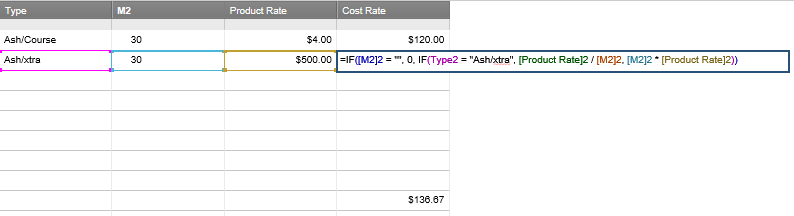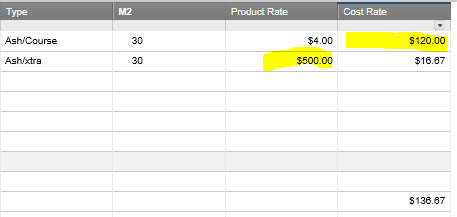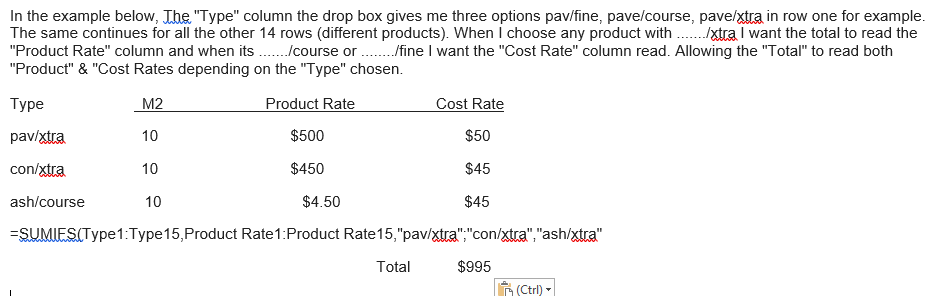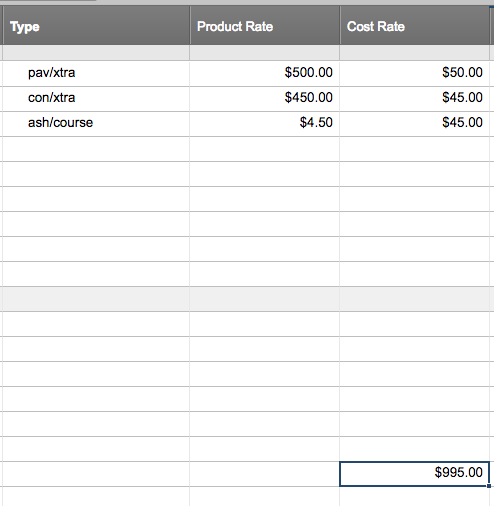# Nested If Statement

Hi Team,

Below is two screen shots. To explain this simply, When I have anything in my drop box with /xtra (Type Column)  I want to have my totaling cell (Bottom of sheet) add up the Product Rate cell in that row.

When its /Course or anything else I want it to add Cost Rate cell in that row.

So my totaling cell (Bottom of sheet) looks something like this: =If([Type]2='Ash/xtra',[Product Rate]2,[Cost Rate]2,If([Type]3="Con/xtra",[Product Rate]3,[Cost Rate]3..............I have a different product on each row, there 14 in total. So ill need a formula that can nest more products as added.

P.s The Ash xtra .PNG attachment is just for the purpose of this exercise (To explain and show)

Each row has its own product, hench the reason for the drop box option for each row.• Hi Heath,

I'd recommend looking at the SUMIF function, instead of an IF statement: https://help.smartsheet.com/function/sumif

This will allow you to summarize values in one column only when the adjacent column contains a certain criterion.

• Hi Shaine,

To get my head around it, could you give me an example please in my situation. Had a couple of goes with no luck ...Cheers

• Screen shot attached explains• Hi Heath,

If I'm understanding you correctly, you'll want to add multiple SUMIF functions together, using the OR function and @cell parameter—like this:

=SUMIF(Type1:Type14, OR(@cell = "pav/xtra", @cell = "con/xtra", @cell = "ash/xtra"), [Product Rate]1:[Product Rate]14) + SUMIF(Type1:Type14, OR(@cell = "ash/course", @cell = "ash/fine"), [Cost Rate]1:[Cost Rate]14)• Thanks again Shaine.

• Hello Heath,

In looking through this and the other post you have on this, I was able to find a more simplistic formula to do what you're looking for. Shaine's formula is great and will work for this, however with the number of values that are possible in your dropdown the formula will get fairly long.

The formula I found returns the same result, however it's slightly more efficient. This also uses the @cell feature, and more on @cell can be found here if needed (https://help.smartsheet.com/articles/2476491#cell). Here's how the formula could be written:

=SUMIF(Type:Type, FIND("xtra", @cell) > 0, [Product Rate]:[Product Rate]) + SUMIF(Type:Type, OR(FIND("course", @cell) > 0, FIND("fine", @cell) > 0), [Cost Rate]:[Cost Rate])

This formula is using the FIND function in combination with @cell (https://help.smartsheet.com/function/find). The FIND function will look at a cell and return the starting position (the number of characters in) of a string within text. In the first SUMIF the FIND function is looking for the string "xtra", and if that string is in the cell the number will be greater than 0 and it will sum the "Product Rate". The second SUMIF does the same thing however it's looking for the string "course" or "fine" and summing the "Cost Rate" column.